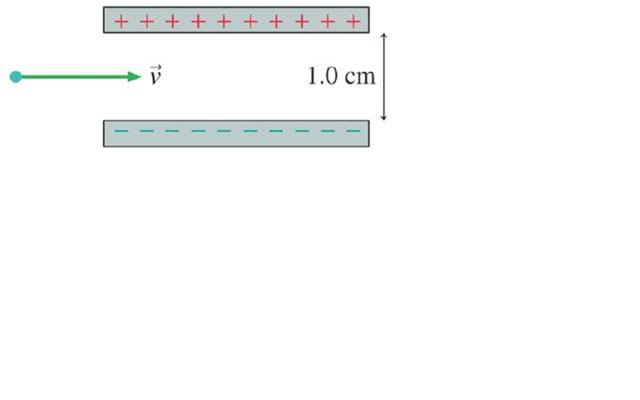# Magnetic Field, Potential, Velocity

## Homework Statement

An electron travels with speed 1.0 x 107
m/s between the two parallel charged
plates shown in the adjacent figure. The
plates are separated by 1.0 cm and are
charged by a 200 V battery. What magnetic
field strength and direction will allow the
electron to pass between the plates without
being deflected?

## Homework Equations

B=($$\mu$$/4$$\pi$$)(qvsin$$\theta$$/r$$^{2}$$)

## The Attempt at a Solution

well im pretty sure that F=0N, and the the direction the field has to be in the same direction of the velocity, so sin(theta)=0...... but i have no idea how find the field strength, anyone have any ideas???

wat can i do with this Voltage... i need a current dont i?... i cant find the right formulas, and getting really frustrated.. argh

Defennder
Homework Helper
The question referred to a picture. It may require a picture or at least an accurate description before we can help. Is the electron travelling in a direction parallel to the plates?

yes sorry... the electron is traveling parallel through the parallel plates.. i see if i can upload pic

Defennder
Homework Helper
Well if that's the case, no picture is required. Just think in terms of how much force is needed to counter-balance the force exerted on the particle by the E-field. Then use the Lorentz force equation to find the magnetic flux density needed.

oh i dont know if we are meant to do it like that, because we havnt done flux or lorentz....Defennder
Homework Helper
Well, "magnetic flux density" is another word for "magnetic field strength" and "Lorentz force" is a more general term for "force on charged particle due to B-field".

oh ok so F=(E+v X B)..... i dont understand because doesnt f have to equal 0, for the electron to pass through undisturbed, as soon as there is a force the electron is going to change direction??? unless its opposing the velocity, which would make it slow down????

thus E=V/s=200/.01=2000N/C but how do i find B?

Defennder
Homework Helper
oh ok so F=(E+v X B)..... i dont understand because doesnt f have to equal 0, for the electron to pass through undisturbed, as soon as there is a force the electron is going to change direction??? unless its opposing the velocity, which would make it slow down????
You're missing out q here. F has to be zero in order for the electron to pass undeflected. Note that the force exerted by the E-field and that by the B-field is perpendicular to its velocity, and hence does not affect its speed in that direction.

thus E=V/s=200/.01=2000N/C but how do i find B?
Use the equation you stated earlier. Although another similar approach would be to separate the two equations: F=qE and F=Bqv and this two are acting in opposite directions, so you equate them and solve for B. Note that you have to indicate the direction in which the B-field is applied.

yes sorry i missed q... so qE=Bqv =>E=Bv=>B=E/v=2000/1x10^7=2x10^-4T in the direction of the velocity?

Defennder
Homework Helper
Check your value for E-field. And note note that magnetic force will always act in a direction perpendicular to both the velocity and direction of the B field. Use the right-hand rule to get the direction.

ya its 20000 not 2000.... oh ok yes i remember now.... duh... lol so the direction of B will be out of the page? since the E travels + to -....

Defennder
Homework Helper
Remember this is an electron, not a positive charged particle.

na im confused... what does this mean... wont the electron want to move towards the positive charged plate??

oh for an electron its the exact opposite, so use left hand, so its going into the page? but is my maths correct, does 2mT sound right?

no wait before i was using my left hand ad it was saying out.... my right says in, thereofre it must be out of the page???? plz help im really confused

Defennder
Homework Helper
No I meant to say that when you apply the F=qv X B vector equation you must note that the resulting direction using the right-hand rule holds for a positive charge. The negative charge goes in the opposite direction.

Defennder
Homework Helper
Don't use your left hand. Your right hand would do, just reverse the direction.

yes so if my right hand says the direction is into the page for a +ve charge it is out the page for a -ve charge ie, an electron?

Defennder
Homework Helper
EDIT: Ok, you're right on this. You can visualise the +ve charged particle as moving from the right to the left. By the way, don't switch it around twice; either consider an equivalent positive charged particle or just reverse the direction at the end of your hand-twisting. Don't do both.

Just consider a positive charge from left to right (same as the electron). Assume the B-field passes into the page. Use the right hand rule and reverse the direction at the end. Is the result the direction you want? If not, assume instead the B-field passes out of the page and do the same.

Last edited: